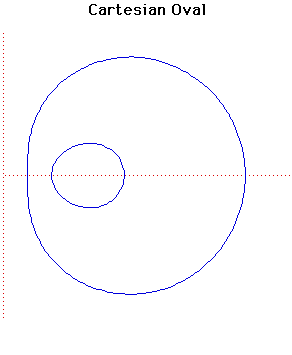# Curves

### Cartesian OvalCartesian equation:
$((1 - m^{2})(x^{2} + y^{2}) + 2m^{2}cx + a^{2} - m^{2}c^{2})^{2} = 4a^{2}(x^{2} + y^{2})$

### Description

This curve $C$ consists of two ovals so it should really be called Cartesian Ovals. It is the locus of a point $P$ whose distances $s$ and $t$ from two fixed points $S$ and $T$ satisfy $s + mt = a$. When $c$ is the distance between $S$ and $T$ then the curve can be expressed in the form given above.

The curves were first studied by Descartes in 1637 and are sometimes called the 'Ovals of Descartes'.

The curve was also studied by Newton in his classification of cubic curves.

The Cartesian Oval has bipolar equation $r + mr' =a$.

If $m = ±1$ then the Cartesian Oval $C$ is a central conic while if $m = a/c$ then the curve is a Limacon of Pascal (Étienne Pascal). In this case the inside oval touches the outside one.

Cartesian Ovals are anallagmatic curves.

### Associated Curves

Definitions of the Associated curves